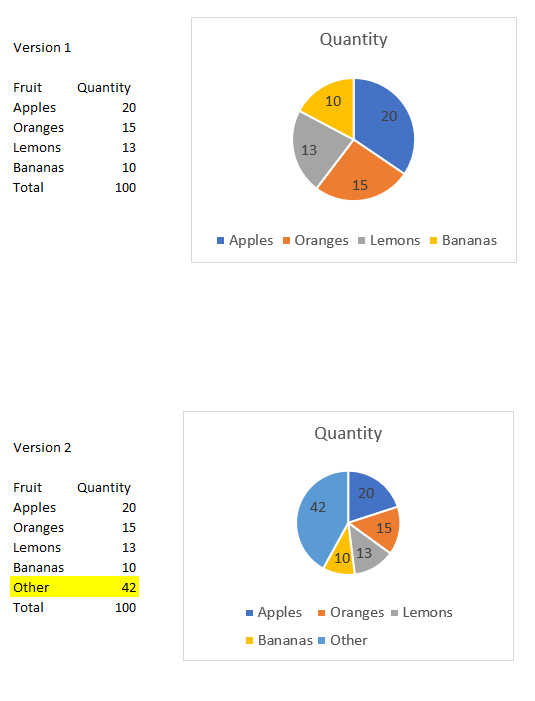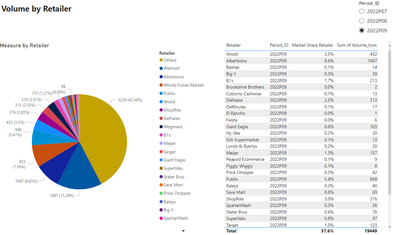cancel
Showing results for
Did you mean:Regular Visitor

## Other category in pie chart

Hello,
I would like to add "other" category to a pie chart. The existing categories in the dataset do not add up to the total, hence "other" needs to be calculated. The illustration below illustrates what I am dealing with (Version 1) and what I would like to accomplish (Version 2). Any ideas on how to do this?1 ACCEPTED SOLUTIONCommunity Support

Hi @SindijaL ,

First create a new table:Then apply the measure to the visual:

``````Measure =
var _a = CALCULATE(SUM(Data[Volume_tons]),FILTER('Data',[Retailer]=MAX('Table'[Retailer])))
var _b = CALCULATE(SUM(Data[Volume_tons]),FILTER('Data',[Retailer]<>"Total"))
var _c = CALCULATE(SUM(Data[Volume_tons]),FILTER('Data',[Retailer]="Total"))
return IF(MAX('Table'[Retailer])="Others",_c-_b,_a)``````

Final output:Best Regards,

Jianbo Li

If this post helps, then please consider Accept it as the solution to help the other members find it more quickly.

3 REPLIES 3Community Support

Hi @SindijaL ,

First create a new table:Then apply the measure to the visual:

``````Measure =
var _a = CALCULATE(SUM(Data[Volume_tons]),FILTER('Data',[Retailer]=MAX('Table'[Retailer])))
var _b = CALCULATE(SUM(Data[Volume_tons]),FILTER('Data',[Retailer]<>"Total"))
var _c = CALCULATE(SUM(Data[Volume_tons]),FILTER('Data',[Retailer]="Total"))
return IF(MAX('Table'[Retailer])="Others",_c-_b,_a)``````

Final output:Best Regards,

Jianbo Li

If this post helps, then please consider Accept it as the solution to help the other members find it more quickly.Solution Sage

@SindijaL Can you explain in the first version how the total is more? without any added rows/values?

if you can share sample data, I might be able to help you better.Regular Visitor

Hi,

Please see the example here: PowerBi Chart

This is the format I receive the data in directly from the source. Multiple retailers are included, as well as a "Total" value for each time period. "Total" does not equal the sum of available retailers because there are other ertailers that are included in "Total" but not stated. I would like to track the market share of each retailer. Currently the sum of available retailers adds up to 57.8% of "Total", implying that "Others" account for 42.2%. I would like to create this "Other" category and display it in the pie chart alongside available retailers.